Get inspired by the success stories of our students in IIT JAM MS, ISI  MStat, CMI MSc DS.  Learn More

# ISI MStat PSB 2012 Problem 10 | MVUE Revisited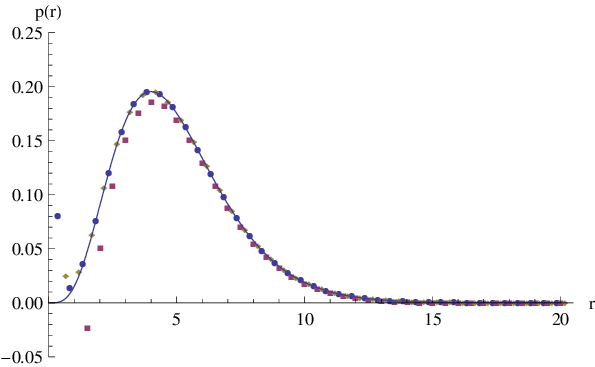This is a very simple sample problem from ISI MStat PSB 2012 Problem 10. It's a very basic problem but very important and regular problem for statistics students, using one of the most beautiful theorem in Point Estimation. Try it!

## Problem- ISI MStat PSB 2012 Problem 10

Let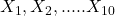be i.i.d. Poisson random variables with unknown parameter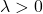. Find the minimum variance unbiased estimator of exp{}.

### Prerequisites

Poisson Distribution

Minimum Variance Unbiased Estimators

Lehman-Scheffe's Theorem

Completeness and Sufficiency

## Solution :

Well, this is a very straight forward problem, where we just need to verify certain conditions, of sufficiency and completeness.

If, one is aware of the nature of Poisson Distribution, one knows that for a given sample, the sufficient statistics for the unknown parameter, is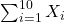, also by extension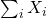is also complete for(How??).

So, now first let us construct an unbiased estimator of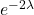. Here, we need to observe patterns as usual. Let us define an Indicator Random variable,,

So,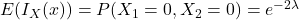, henceis an unbiased estimator of. But is it a Minimum Variance ??

Well, Lehman-Scheffe answers that, Since we know thatis complete and sufficient for, By Lehman-Scheffe's theorem,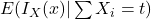is the minimum variance unbiased estimator offor any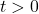. So, we need to find the following,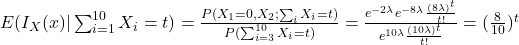.

So, the Minimum Variance Unbiased Estimator of exp{} is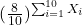Now can you generalize this for a sample of size n, again what if I definedas,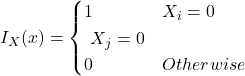, for some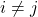,

would it affected the end result ?? What do you think?

## Food For Thought

Let's not end our concern for Poisson, and think further, that for the given sample if the sample mean is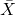and sample variance is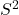. Can you show that, and further can you extend your deductions to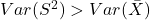??

Finally can you generalize the above result ?? Give some thoughts to deepen your insights on MVUE.

## Subscribe to Cheenta at Youtube

This is a very simple sample problem from ISI MStat PSB 2012 Problem 10. It's a very basic problem but very important and regular problem for statistics students, using one of the most beautiful theorem in Point Estimation. Try it!

## Problem- ISI MStat PSB 2012 Problem 10

Letbe i.i.d. Poisson random variables with unknown parameter. Find the minimum variance unbiased estimator of exp{}.

### Prerequisites

Poisson Distribution

Minimum Variance Unbiased Estimators

Lehman-Scheffe's Theorem

Completeness and Sufficiency

## Solution :

Well, this is a very straight forward problem, where we just need to verify certain conditions, of sufficiency and completeness.

If, one is aware of the nature of Poisson Distribution, one knows that for a given sample, the sufficient statistics for the unknown parameter, is, also by extensionis also complete for(How??).

So, now first let us construct an unbiased estimator of. Here, we need to observe patterns as usual. Let us define an Indicator Random variable,,

So,, henceis an unbiased estimator of. But is it a Minimum Variance ??

Well, Lehman-Scheffe answers that, Since we know thatis complete and sufficient for, By Lehman-Scheffe's theorem,is the minimum variance unbiased estimator offor any. So, we need to find the following,.

So, the Minimum Variance Unbiased Estimator of exp{} isNow can you generalize this for a sample of size n, again what if I definedas,, for some,

would it affected the end result ?? What do you think?

## Food For Thought

Let's not end our concern for Poisson, and think further, that for the given sample if the sample mean isand sample variance is. Can you show that, and further can you extend your deductions to??

Finally can you generalize the above result ?? Give some thoughts to deepen your insights on MVUE.

## Subscribe to Cheenta at Youtube

This site uses Akismet to reduce spam. Learn how your comment data is processed.

### Knowledge Partner